The VARCLUS Procedure
 Computational Resources

Let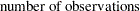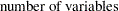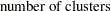It is assumed that, at each stage of clustering, the clusters all contain the same number of variables.

### Time

The time required for the VARCLUS procedure to analyze a given data set varies greatly depending on the number of clusters requested, the number of iterations in both the alternating least-squares and search phases, and whether centroid or principal components are used.

The time required to compute the correlation matrix is roughly proportional to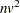.

Default cluster initialization requires time roughly proportional to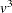. Any other method of initialization requires time roughly proportional to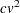.

In the alternating least-squares phase, each iteration requires time roughly proportional toif centroid components are used or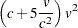if principal components are used.

In the search phase, each iteration requires time roughly proportional to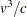if centroid components are used or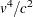if principal components are used. The HIERARCHY option speeds up each iteration after the first split by as much as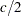.

### Memory

The amount of memory, in bytes, needed by the VARCLUS procedure is approximately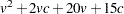Previous Page | Next Page | Top of Page